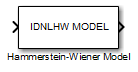Hammerstein-Wiener Model

Simulate Hammerstein-Wiener model in Simulink software

• Library:
• System Identification Toolbox / Models

•Description

The Hammerstein-Wiener Model block simulates the output of a Hammerstein-Wiener model using time-domain input data. The model is an idnlhw model that you previously estimated or constructed in the MATLAB® workspace. You specify initial conditions for the simulation as one of the following:

• Zero for all states

• Initial state vector representing the initial states of the linear block

For information about the structure of a Hammerstein-Wiener model, see What are Hammerstein-Wiener Models?.

Ports

Input

expand all

Simulation input data, specified as a scalar for a single-input model. The data must be time-domain data. For multi-input models, specify the input as an Nu-element vector, where Nu is the number of inputs. For example, you can use a Vector Concatenate (Simulink) block to concatenate scalar signals into a vector signal.

Note

Do not use a Bus Creator (Simulink) or Mux (Simulink) block to produce the vector signal.

Data Types: double

Output

expand all

Simulated output from Hammerstein-Wiener model, returned as a scalar for a single-output model and as an Ny-element vector for a model with Ny outputs.

Data Types: double

Parameters

expand all

Hammerstein-Wiener model to be simulated, specified as an idnlhw object. You previously estimate or construct the idnlhw model in the MATLAB workspace.

The states of a Hammerstein-Wiener model correspond to the states of the embedded linear idpoly or idss model. For more information about the states, see the idnlhw reference page. You specify Initial conditions as one of the following:

• Zero — Specifies zero initial state values, which correspond to a simulation starting from a state of rest.

• State values — You specify the state values in Specify a vector of state values. Specify the states as a vector of length equal to the number of states in the model.

If you do not know the initial states, you can estimate these states as follows:

• To simulate the model around a given input level when you do not know the corresponding output level, you can estimate the equilibrium state values using the findop command.

For example, to simulate a model M about a steady-state point where the input is 1 and the output is unknown, you can specify the initial state values as X0, where:

• To estimate the initial states that provide a best fit between measured data and the simulated response of the model for the same input, use the findstates command.

For example, to compute initial states such that the response of the model M matches the simulated output data in the data set z, specify X0, such that:

X0 = findstates(M,z)

Extended Capabilities

Blocks

Introduced in R2008a

System Identification Toolbox DocumentationGet trial now